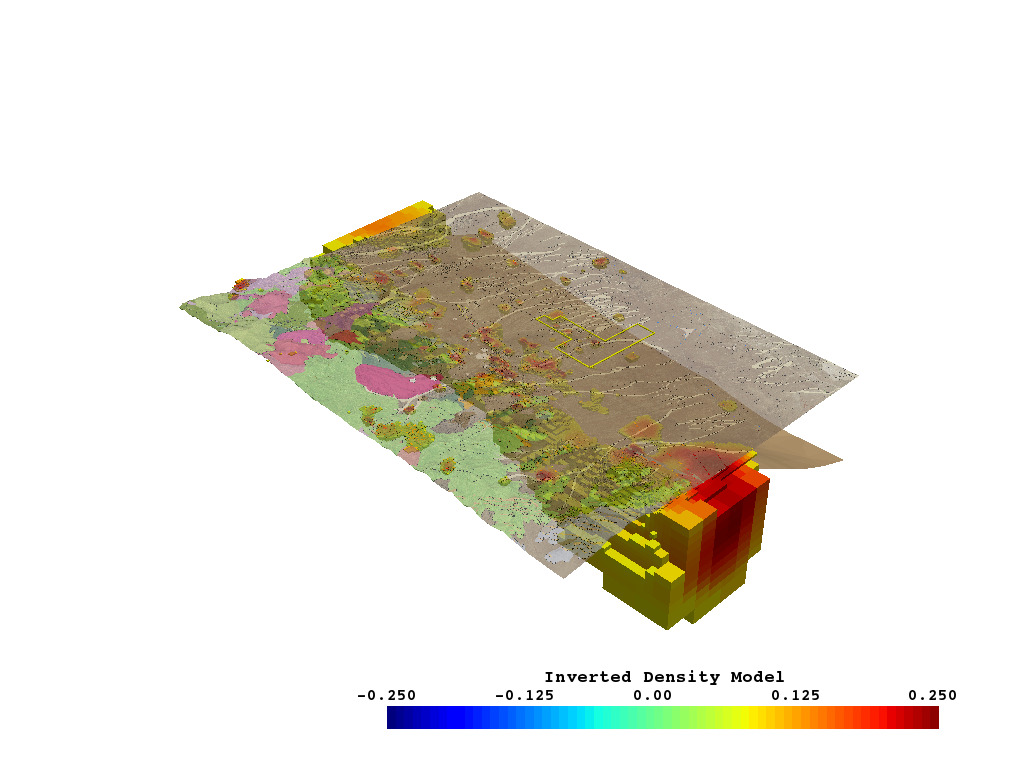# Inspect Gravity Model¶

This notebook examines the inverted gravity model and shows off a useful PVGeo algorithm for rmeove parts of a mesh/model that are baove a topographic surface.

```# sphinx_gallery_thumbnail_number = 6

# Import project package
import gdc19
```
```import pyvista
import PVGeo
import omfvista
import pandas as pd
import numpy as np
```

Load all the datasets created in the data aggreagation section

```gis_data = omfvista.load_project(gdc19.get_project_path('gis.omf'))
print(gis_data.keys())
```

Out:

```['boundary']
```
```surfaces = omfvista.load_project(gdc19.get_project_path('surfaces.omf'))
print(surfaces.keys())
```

Out:

```['land_surface', 'temp_225c', 'temp_175c', 'opal_mound_fault', 'negro_mag_fault', 'top_granitoid']
```

```gf = gdc19.get_gravity_path('forge_inverse_problem/RESULT_THRESHED.vtu')
grav_model.active_scalar_name = 'Magnitude'
```

Grab data from multi blocks for conveinance

```topo = surfaces['land_surface']
granitoid = surfaces['top_granitoid']

p = pyvista.Plotter()
p.show()
```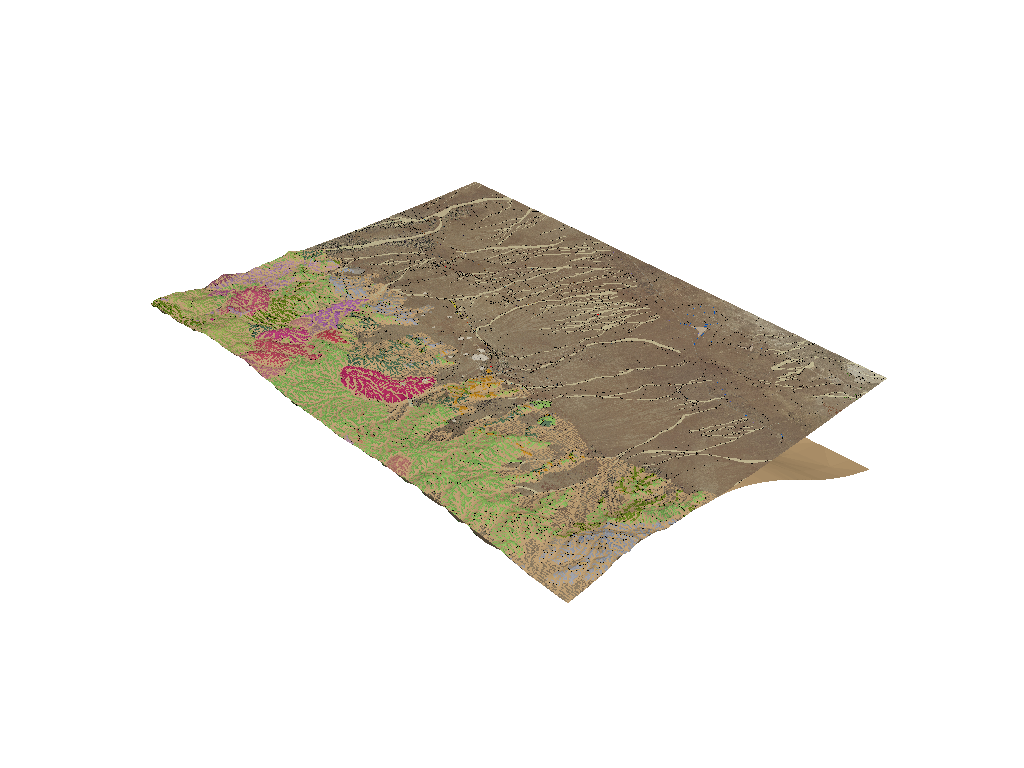Note how the above figure has artificats from where the top of granite surface boundary matches the topographic surface. To mitigate these effect, we can use a filtering technique that will remove parts of a mesh above or within a tolerance of a given surface. PVGeo has a filter that perfroms this type of operation with ease.

Let’s run a PVGeo filter to extract the topo surface from the granitoid surface - `PVGeo.grids.ExtractTopography`

```# Run the PVGeo algorithm
granitoid = PVGeo.grids.ExtractTopography(
remove=True, # remove the inactive cells
tolerance=10.0 # buffer around the topo surface
).apply(granitoid, topo)

p = pyvista.Plotter()
p.show()
```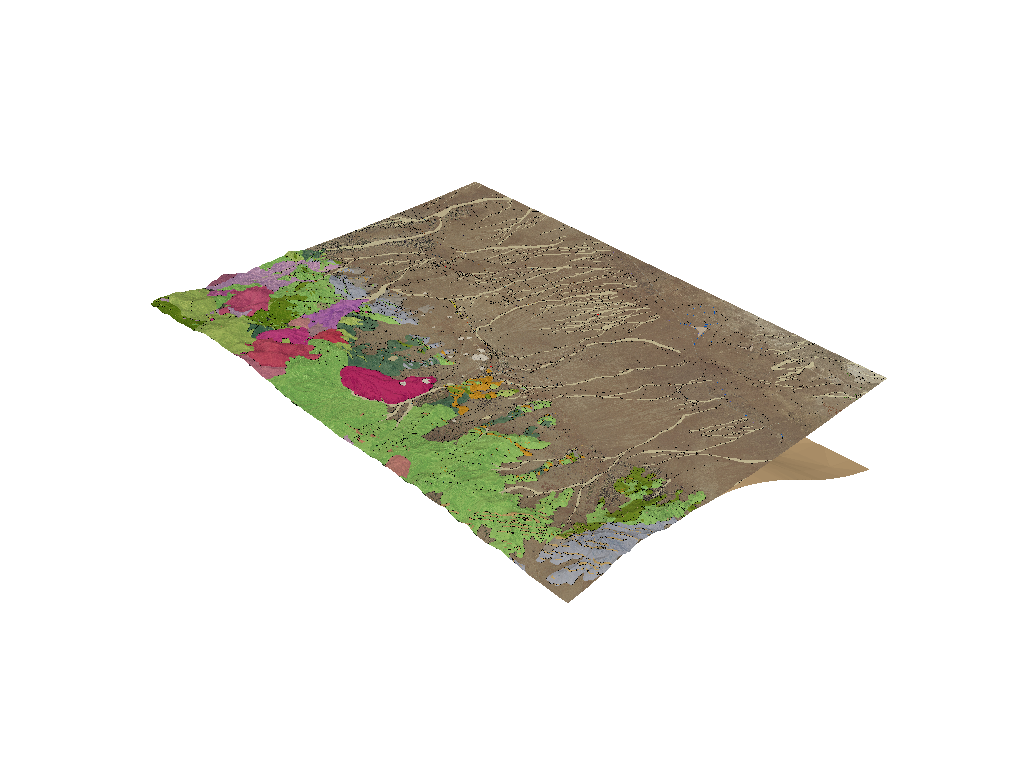Now both the topographic surface and the granitoid boundary can be rendered without arficats due to where they overlap.

This type of topography extraction is often very useful with 3D models where the model domain goes above the topographic surface - thus we may want to parts of a 3D model above the topography. Let’s try this with the gravity model.

```grav_kwargs = dict(
cmap='jet',
clim=[-0.25,0.25],
stitle='Inverted Density Model'
)

temp_kwargs = dict(
cmap='coolwarm',
clim=[0,255],
stitle='Temperature (C)'
)
```

Now extract the topographic surface from the model to have a more realistic domain:

```# Remove values above topography
grav_model_no_topo = PVGeo.grids.ExtractTopography(
remove=True, # remove the inactive cells
tolerance=10.0 # buffer around the topo surface
).apply(grav_model, topo)

grav_model_no_topo.plot(**grav_kwargs)
```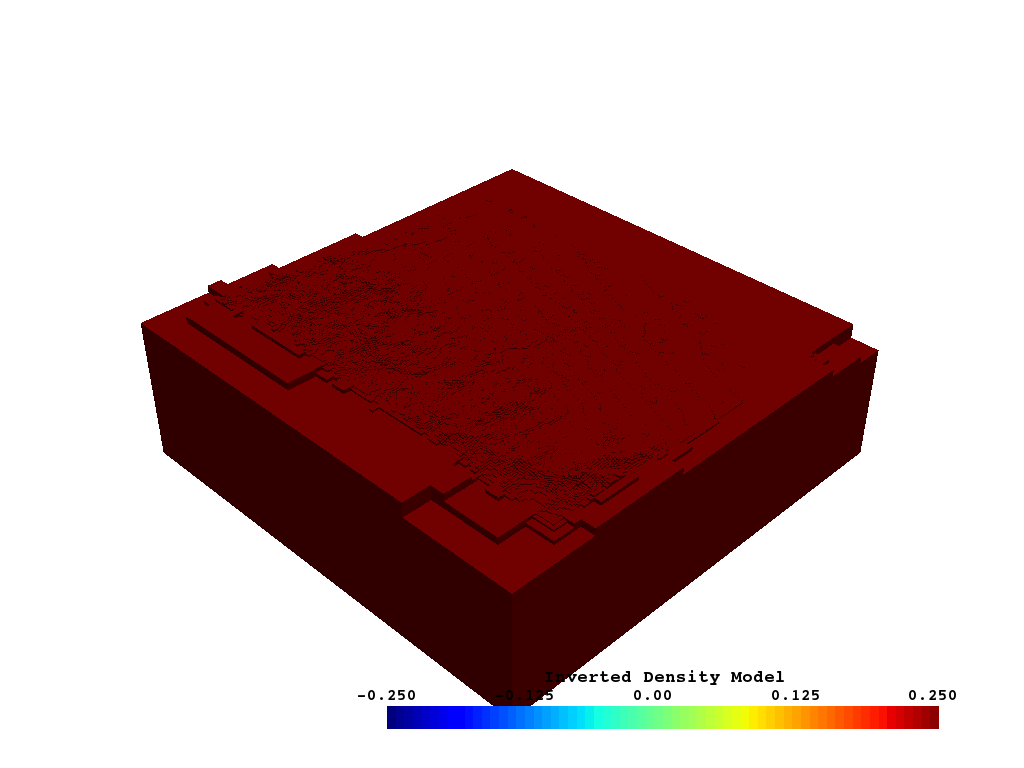```grav_roi = grav_model.threshold(0.07)
grav_roi.plot(**grav_kwargs)
```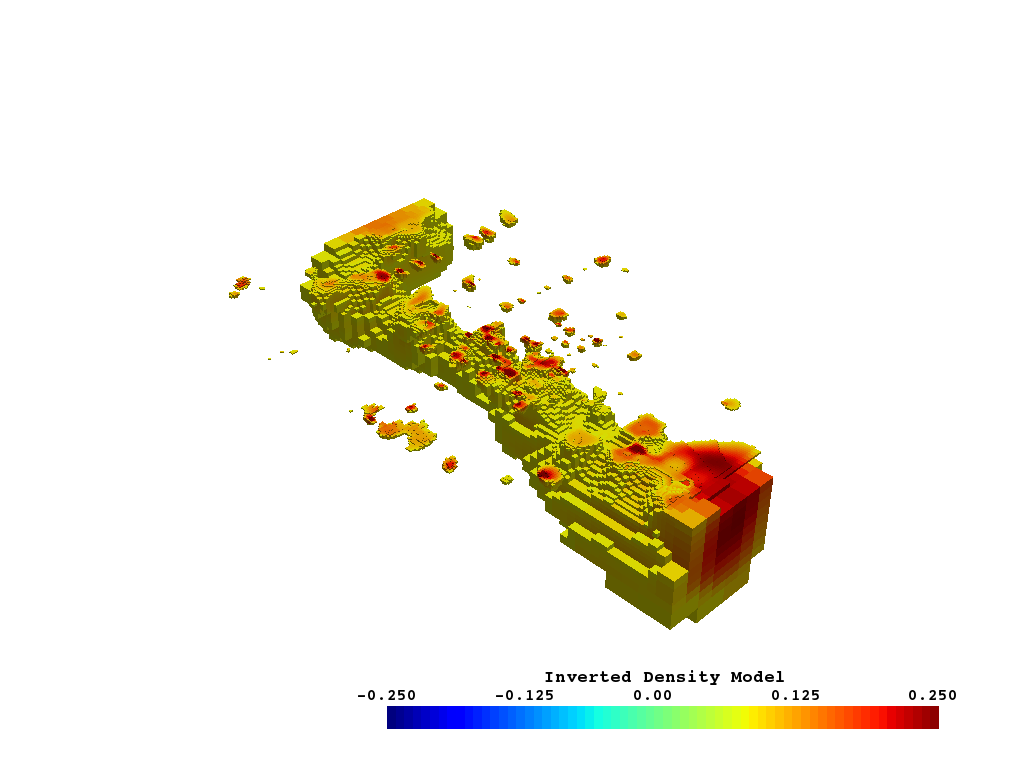And just out of curiosity, how big of a volume is that density range?

```print('Gravity model Region is {:.2f} cubic kilometers.'.format(grav_roi.volume * 1e-9))
```

Out:

```WARNING:root:DEPRECATED: ``.tri_filter`` is deprecated. Use ``.triangulate`` instead.
WARNING:root:DEPRECATED: ``.tri_filter`` is deprecated. Use ``.triangulate`` instead.
Gravity model Region is 167.14 cubic kilometers.
```

How do the provided temperature surface contours look next to the inverted gravity model?

```temp_175c = surfaces['temp_175c']
temp_225c = surfaces['temp_225c']

p = pyvista.Plotter()
p.show()
```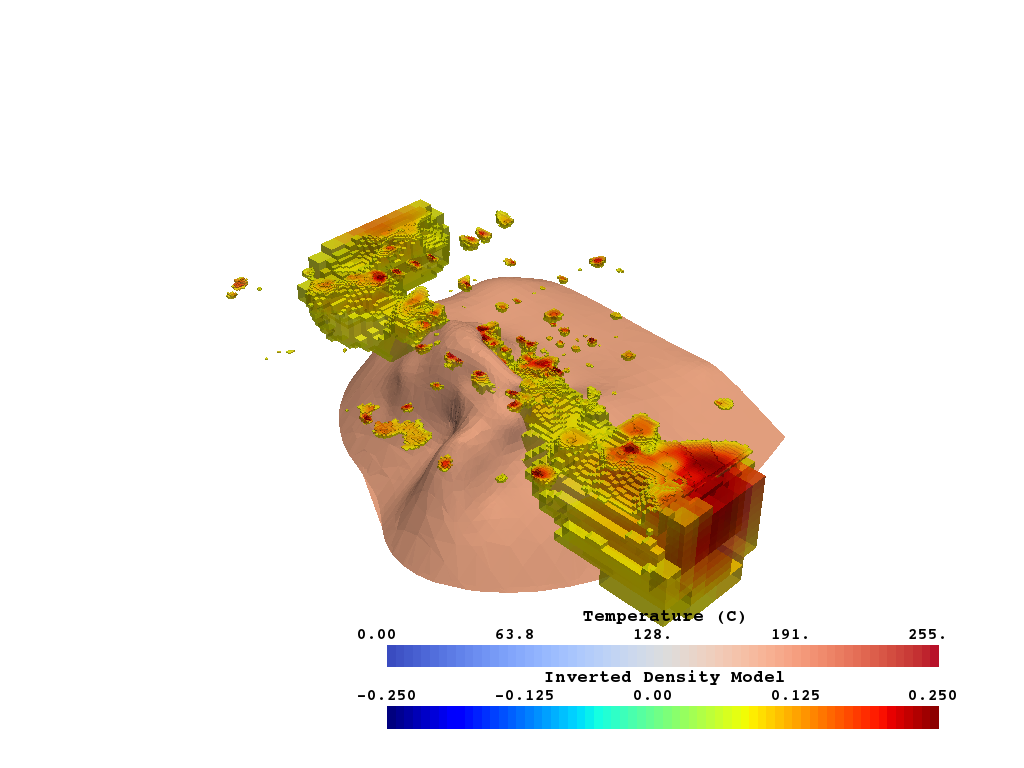Now lets put this all together to gain insight on where that dense body is in relation to the FRGE site

```boundary = gis_data['boundary']